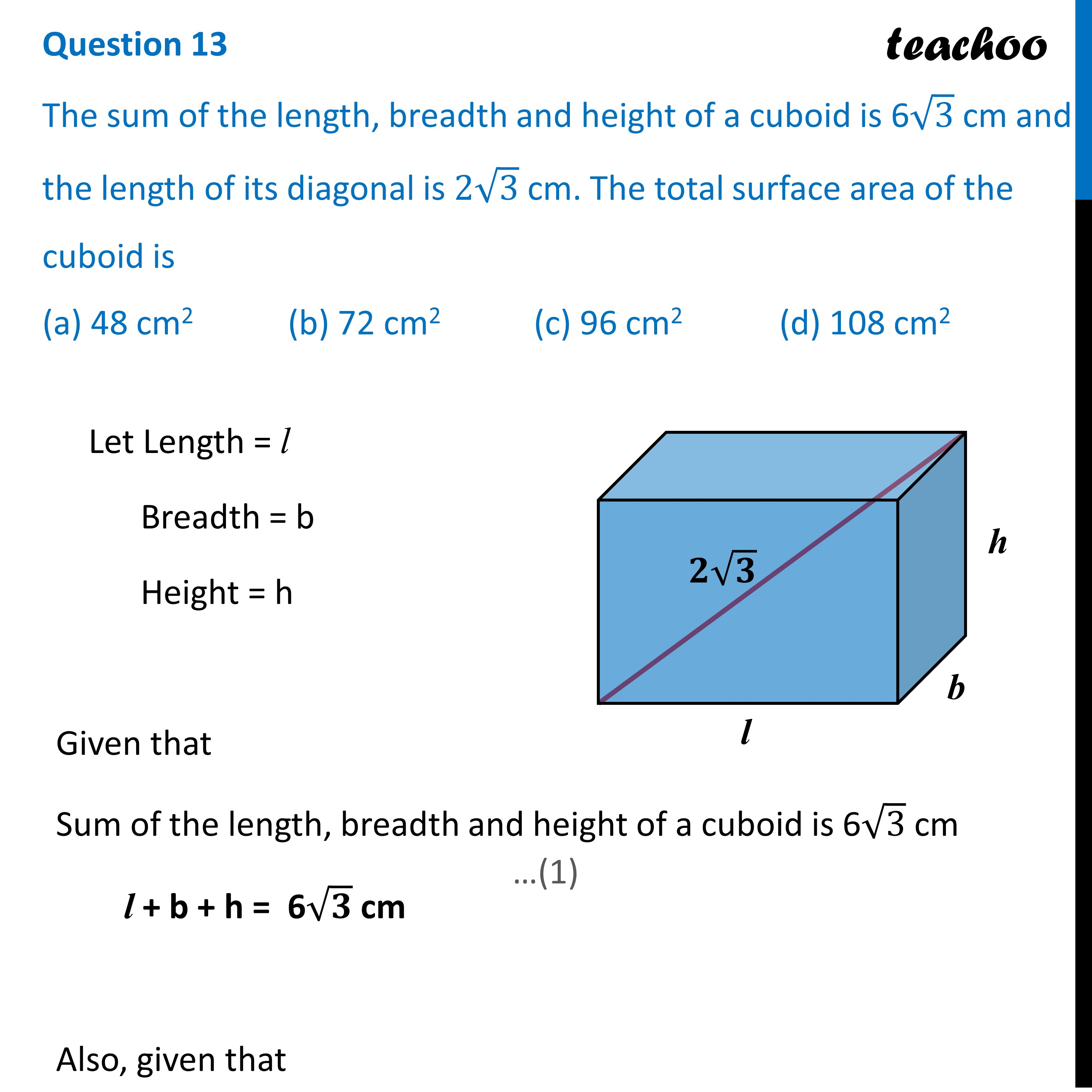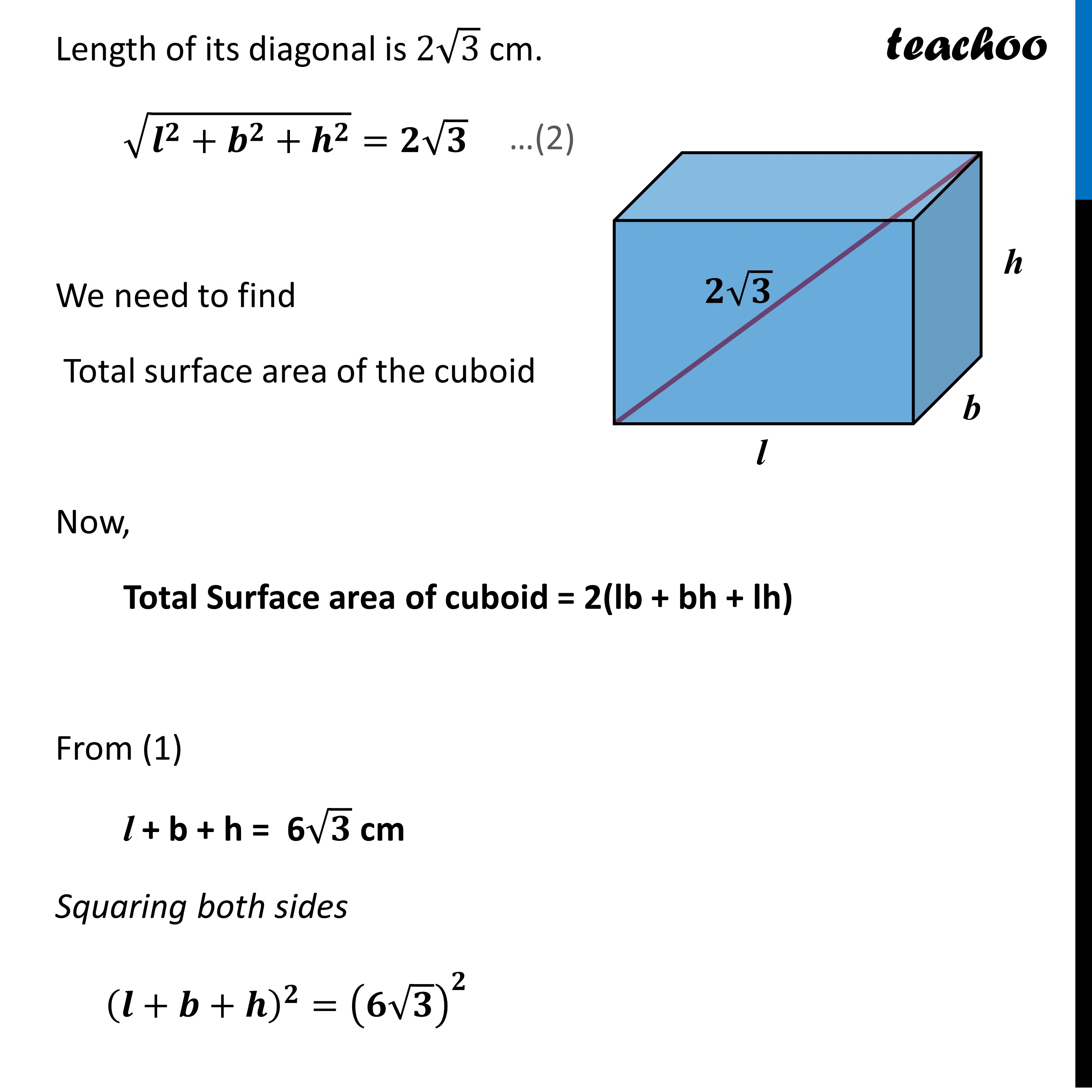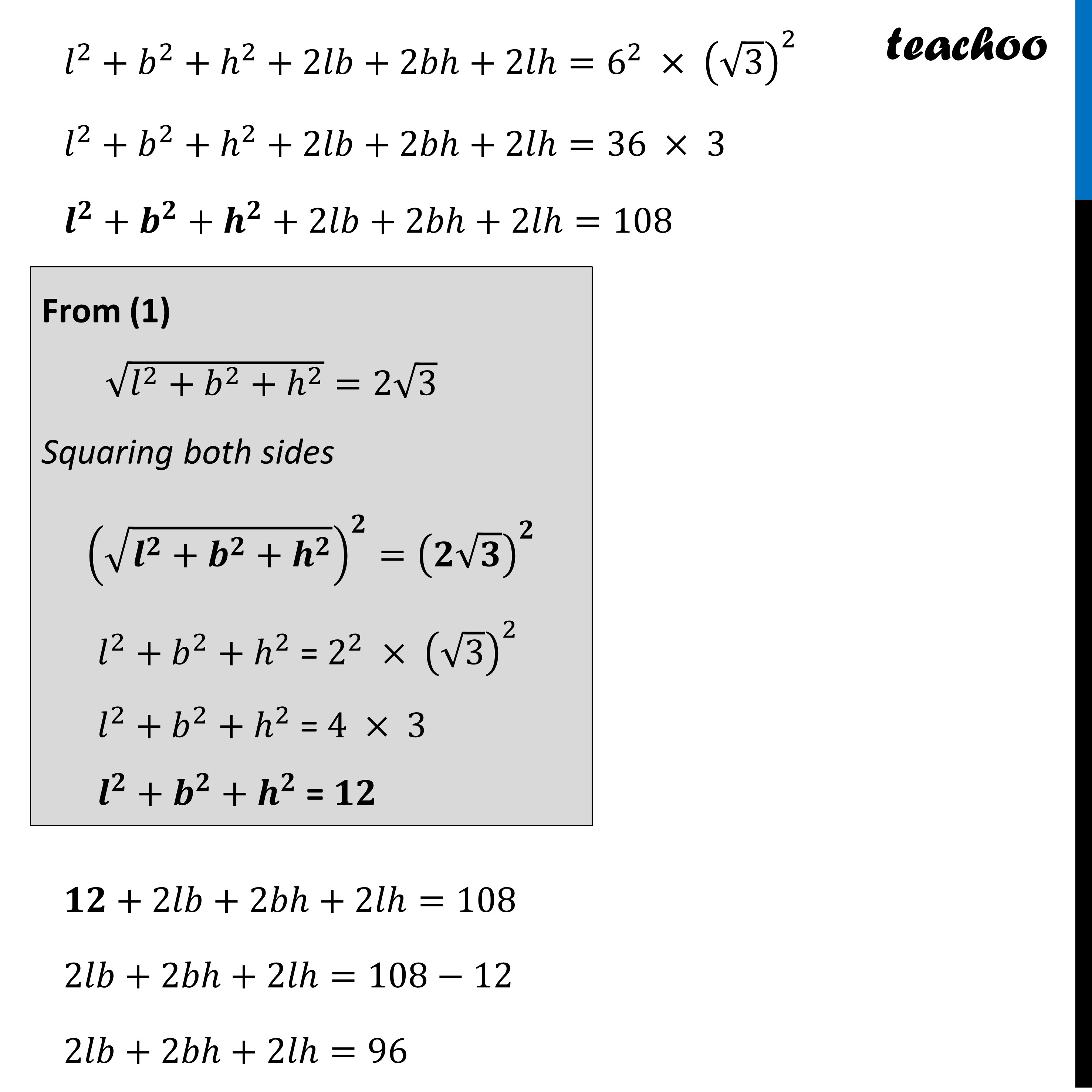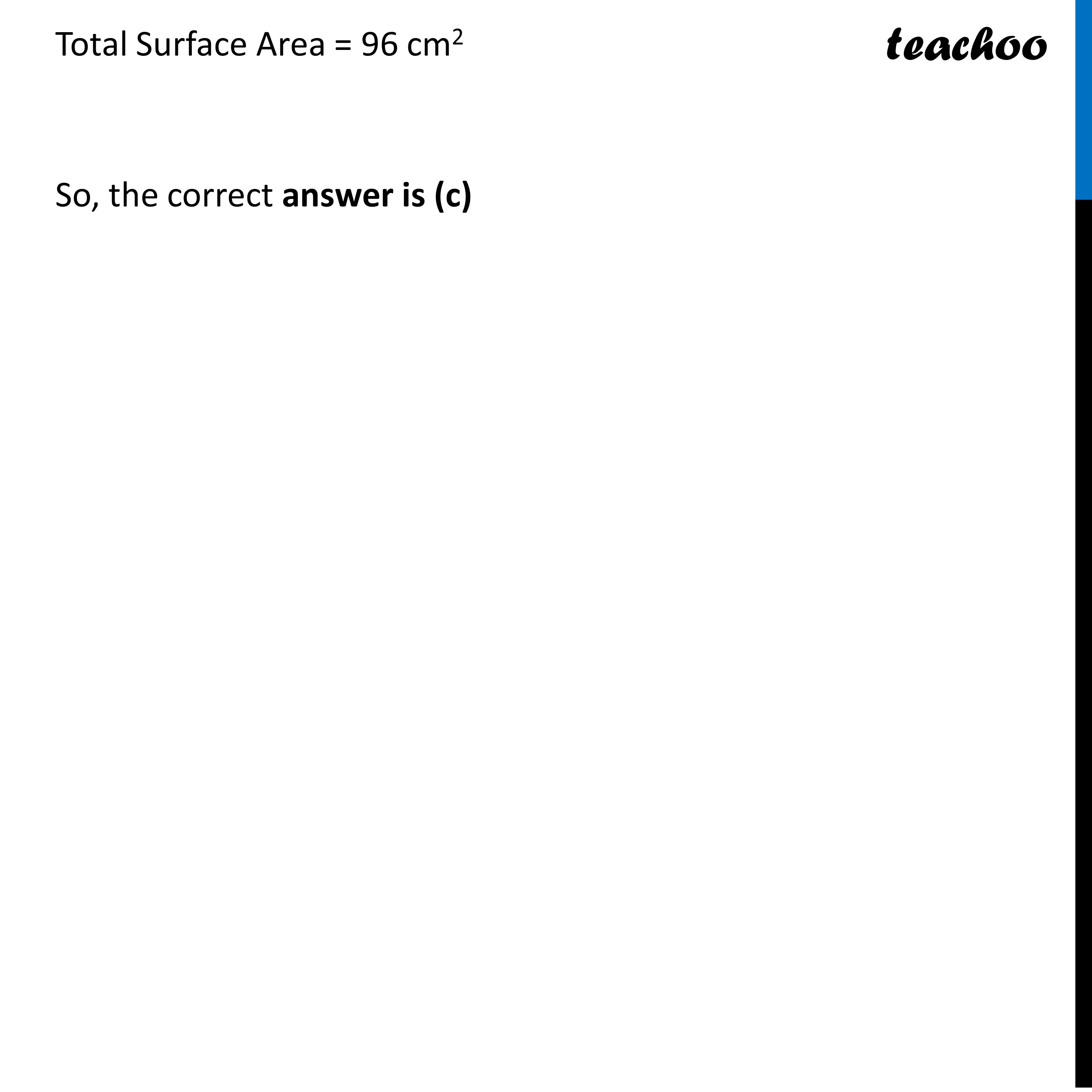CBSE Class 10 Sample Paper for 2023 Boards - Maths Standard

Class 10
Solutions of Sample Papers for Class 10 Boards

## (a) 48 cm 2   (b) 72 cm 2   (c) 96 cm 2   (d) 108 cm 2Learn in your speed, with individual attention - Teachoo Maths 1-on-1 Class

### Transcript

Question 13 The sum of the length, breadth and height of a cuboid is 6√3 cm and the length of its diagonal is 2√3 cm. The total surface area of the cuboid is (a) 48 cm2 (b) 72 cm2 (c) 96 cm2 (d) 108 cm2 Let Length = l Breadth = b Height = h Given that Sum of the length, breadth and height of a cuboid is 6√3 cm l + b + h = 6√𝟑 cm Also, given that Length of its diagonal is 2√3 cm. √(𝒍^𝟐+𝒃^𝟐+𝒉^𝟐 )=𝟐√𝟑 We need to find Total surface area of the cuboid Now, Total Surface area of cuboid = 2(lb + bh + lh) From (1) l + b + h = 6√𝟑 cm Squaring both sides (𝒍+𝒃+𝒉)^𝟐=(𝟔√𝟑)^𝟐 𝑙^2+𝑏^2+ℎ^2+2𝑙𝑏+2𝑏ℎ+2𝑙ℎ=6^2 × (√3)^2 𝑙^2+𝑏^2+ℎ^2+2𝑙𝑏+2𝑏ℎ+2𝑙ℎ=36 × 3 𝒍^𝟐+𝒃^𝟐+𝒉^𝟐+2𝑙𝑏+2𝑏ℎ+2𝑙ℎ=108 𝟏𝟐+2𝑙𝑏+2𝑏ℎ+2𝑙ℎ=108 2𝑙𝑏+2𝑏ℎ+2𝑙ℎ=108−12 2𝑙𝑏+2𝑏ℎ+2𝑙ℎ=96 𝑙^2+𝑏^2+ℎ^2+2𝑙𝑏+2𝑏ℎ+2𝑙ℎ=6^2 × (√3)^2 𝑙^2+𝑏^2+ℎ^2+2𝑙𝑏+2𝑏ℎ+2𝑙ℎ=36 × 3 𝒍^𝟐+𝒃^𝟐+𝒉^𝟐+2𝑙𝑏+2𝑏ℎ+2𝑙ℎ=108 𝟏𝟐+2𝑙𝑏+2𝑏ℎ+2𝑙ℎ=108 2𝑙𝑏+2𝑏ℎ+2𝑙ℎ=108−12 2𝑙𝑏+2𝑏ℎ+2𝑙ℎ=96 Total Surface Area = 96 cm2 So, the correct answer is (c)